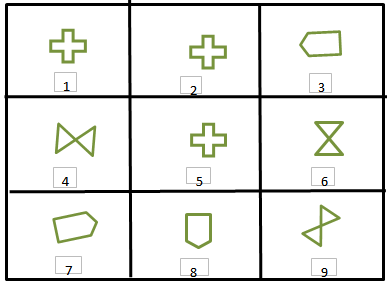# Indian Talent Olympiad - IMO PDF Sample Papers for Class 9

Class 9 sample paper & practice questions for International Maths Olympiad (IMO) level 1 are given below. Syllabus for level 1 is also mentioned for these exams. You can refer these sample paper & quiz for preparing for the exam.#### Resources:

##### Sample Questions from Olympiad Success:
 Q.1 Q.2 Q.3 Q.4 Q.5 Q.6 Q.7 Q.8 Q.9 Q.10
 Q.1 The least number which when divided by 35, leaves remainder of 25, when divided with 45 leaves a remainder of 35 and when divided by 55 leaves 45 as remainder, is: a) 3455 b) 3465 c) 3475 d) 10
 Q.2 If surface area of sphere is 144π cm². What is its radius? a) 5 cm b) 6 cm c) 7 cm d) 8 cm
 Q.3 If a rectangular tank is 21 cm long, 13 cm wide and 18 cm high and contains water up to a height of 11 cm then volume of water in liters is: a) 4.003 liters b) 5.003 liters c) 3.003 liters d) 2.003 liters
 Q.4 In ΔPQR, ∠P = 60°, ∠Q = 50°. Which side of the triangle is the longest? a) PQ b) QR c) PR d) None of these
 Q.5 What is the probability of getting a total of less than 12 in the throws of two dice? a) 35/36 b) 21/36 c) 2/6 d) 3/8
 Q.6 If BAD is written as YZW and SAME as HZNV, then LOVE will be coded as: a) ROWN b) OJUC c) OLEV d) NOPL
 Q.7 Identify the identical figures from the following options.a) (1,2,5), (3,8,9), (4,6,7) b) (1,2,5), (4,7,8), (5,6,9) c) (1,2,5), (3,7,8), (4,6,9) d) (1,3,5), (2,7,8) (4,6,9)
 Q.8 If a cuboid is 2.7 cm high, 7.8 cm long and 3.7 cm wide then volume of cuboid is? a) 77.922 cm3 b) 82.987 cm3 c) 83.456 m3 d) 85.687 cm3
 Q.9 A number is as much greater than 22 as is less than 72. Then the number is: a) 47 b) 50 c) 52 d) 104
 Q.10 The value of x such that AB = BC where A = (6, -1), B = (1, 3) and C = (x, 8) is: a) 5 or 0 b) 4 or -2 c) 5 or -3 d) None of the aboveSample PDF of Indian Talent Olympiad - International Maths Olympiad (IMO) PDF Sample Papers for Class 9:

 Q.1 )a Q.2 )b Q.3 )c Q.4 )a Q.5 )a Q.6 )c Q.7 )c Q.8 )a Q.9 )a Q.10 )c

Q.1 : a | Q.2 : b | Q.3 : c | Q.4 : a | Q.5 : a | Q.6 : c | Q.7 : c | Q.8 : a | Q.9 : a | Q.10 : c